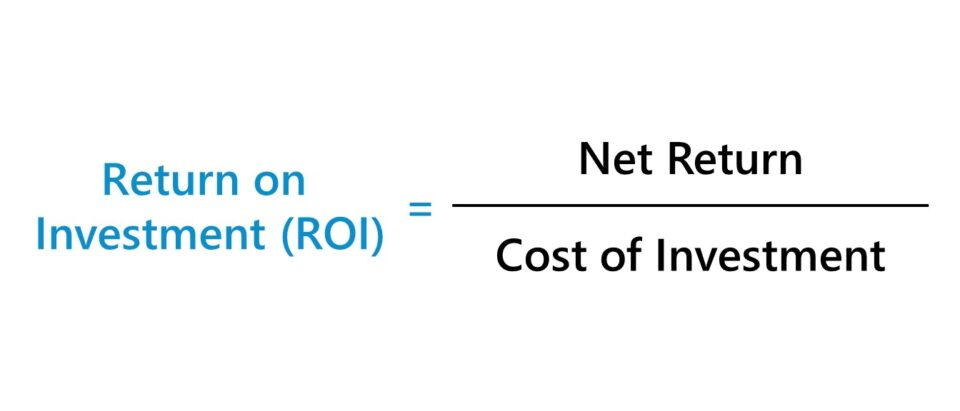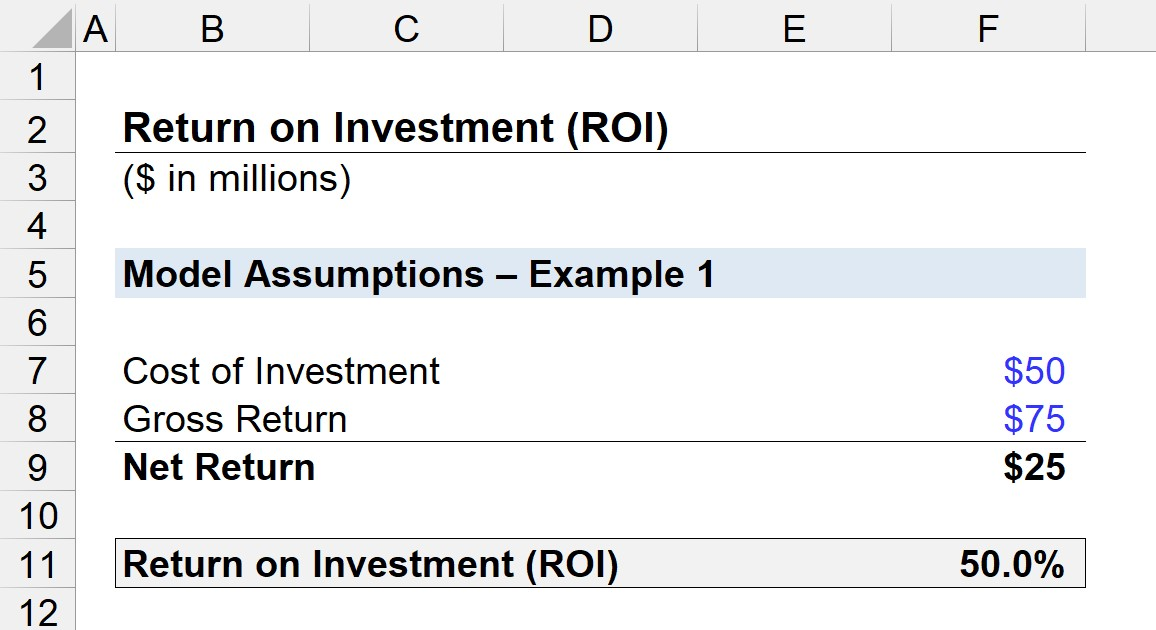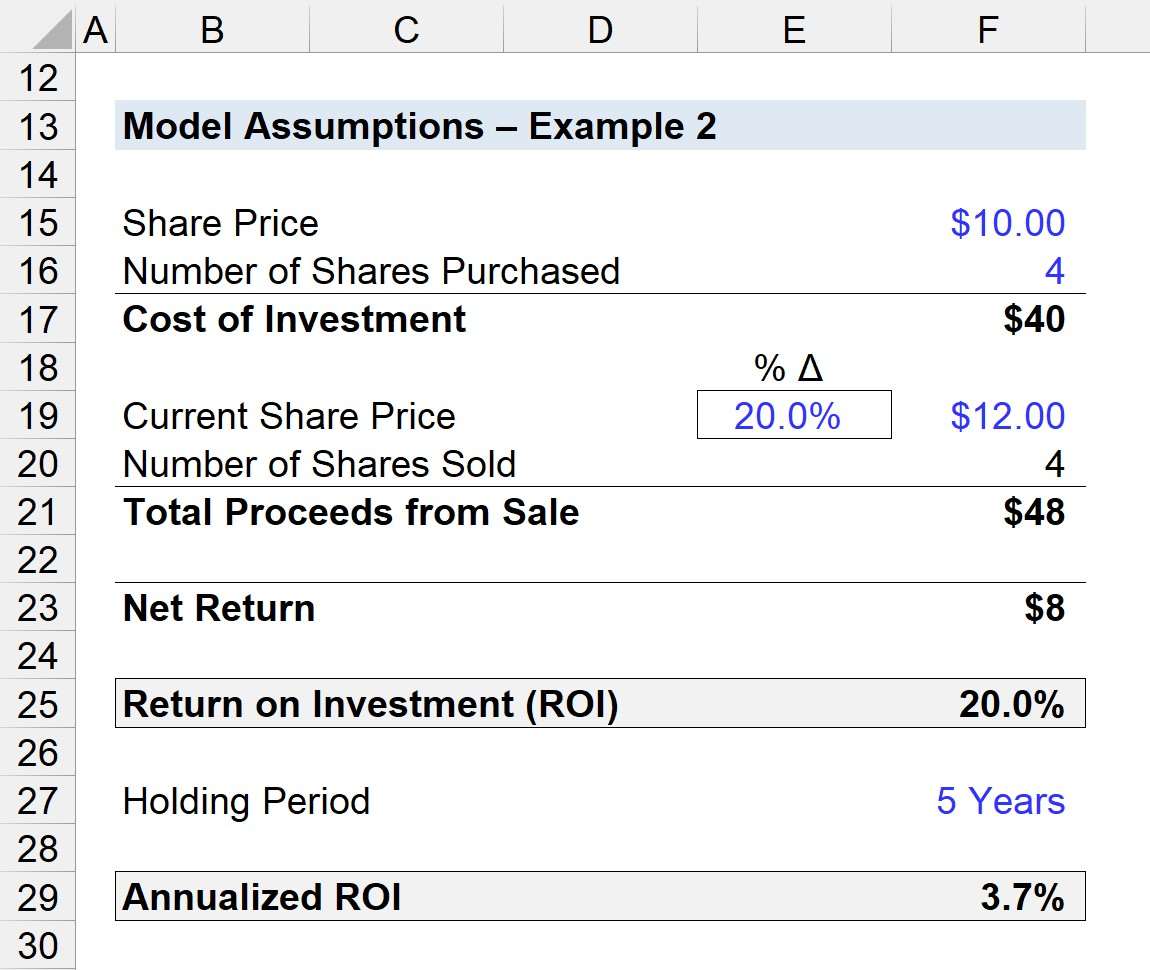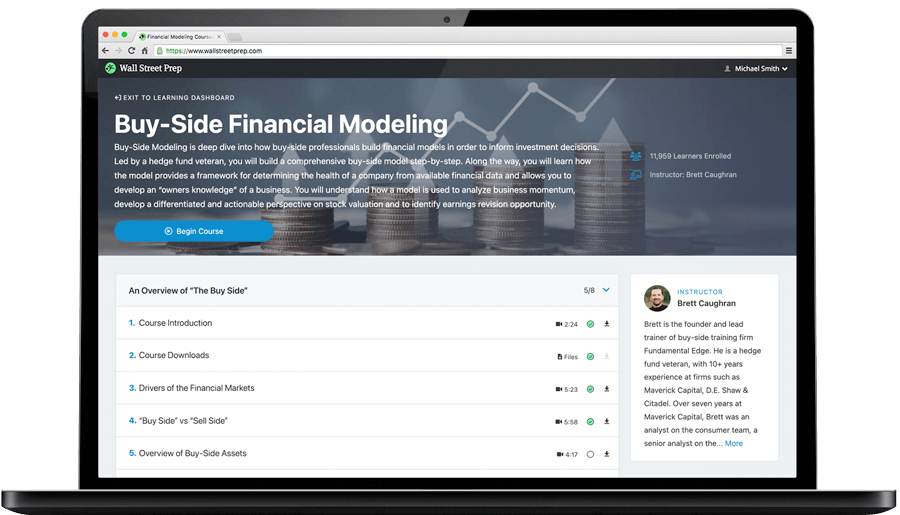Welcome to Wall Street Prep! Use code at checkout for 15% off.# Return on Investment (ROI)

Guide to Understanding Return on Investment (ROI)• ROI stands for “Return on Investment” and measures the profitability of an investment relative to its cost.
• ROI is a method for businesses and investors to determine the efficiency and effectiveness of their capital allocation and investment strategy.
• The ROI formula is the ratio between the net profit earned on an investment and the cost of the investment, expressed as a percentage.
• The ROI ratio is a practical tool for investors because the metric can guide informed financial decisions, such as for tracking the performance of existing investments, or to evaluate the potential return of an investment.

## How to Calculate ROI?

The ROI, an abbreviation for “return on investment”, is the ratio between the net return and the cost of an investment.

The return on investment (ROI) formula is straightforward, as the calculation simply involves dividing the net return on the investment by the investment’s corresponding cost.

• Net Return → Total Profits Received
• Cost of the Investment → Total Amount Spent

In practice, the ROI is not only used by individual and institutional investors to track their portfolio, but also by corporations that rely on the metric for internal purposes, such as their decision-making processes regarding which projects to pursue and for decisions on optimizing how to allocate their capital on hand.

Therefore, the ROI is a method for investors to determine the efficiency of their current capital allocation strategy, including the effectiveness of past investments.

The higher the return on investment (ROI) on a project or investment, the greater the monetary benefits received — all else being equal.

However, what constitutes whether the ROI is adequate differs based on the target return specific to the investor and the length of the holding period, among other factors.

## ROI Formula

The formula for calculating the return on investment (ROI) is as follows.

Return on Investment (ROI) = (Gross Return Cost of Investment) ÷ Cost of Investment

The difference between the gross return and the cost of investment is the net return.

Return on Investment (ROI) = Net Return ÷ Cost of Investment

For purposes of comparability, the return on investment metric is typically expressed in percentage form, so the resulting value from the above formula must then be multiplied by 100.

The numerator in the formula, the return, represents the “net” return — meaning that the cost of the investment must be subtracted from either:

1. Gross Returns (or)
2. Total Exit Proceeds

## Quick ROI Calculation Example

For example, if the gross return on an investment is \$100k while the associated cost was \$80k, the net return is \$20k.

• Gross Return = \$100k
• Initial Cost = \$80k
• Net Return = \$20k

With that said, the return on investment (ROI) ratio can be calculated by dividing the \$20k net return by the cost of \$80k, which comes out to 25%.

• Return on Investment (ROI) = \$20k ÷ \$80k = 0.25, or 25%

## What is a Good ROI Ratio?

The return on investment (ROI) is a widespread financial metric due to its simplicity, since only two inputs are necessary to calculate the ratio.

1. Net Return
2. Cost of Investment

However, one drawback is that the “time value of money” is neglected, i.e. a dollar received today in worth more than a dollar received in the future.

If there are two investments with the same return, yet the second investment requires twice the amount of time until it is realized, the ROI metric on its own fails to capture this important distinction.

Therefore, when making comparisons among different investments, investors must ensure the time frame is the same (or nearby) or otherwise remain cognizant of the timing discrepancies between investments when putting together rankings.

One variation of the metric is called the annualized return on investment, which adjusts the metric for differences in timing.

Annualized ROI = [(Ending Value ÷ Beginning Value) ^ (1 ÷ Number of Years)] 1

Furthermore, a common mistake in calculating the metric is neglecting side expenses, which tends to be more applicable to projects in corporate finance.

The ROI calculation must factor in each and every profit and incurred cost associated with the project (e.g. unexpected maintenance fees) and investments (e.g. dividends, interest).

## ROI Calculator

We’ll now move to a modeling exercise, which you can access by filling out the form below.#### Excel TemplateSubmitting...

## 1. ROI Calculation Example

Suppose an industrial company spent \$50 million in capital expenditures (Capex) to invest in new machinery and upgrade their factory.

By the end of the anticipated holding period – which in the context of a company purchasing fixed assets is the end of the PP&E’s useful life assumption – the company received \$75 million.

The net return on the PP&E investment is equal to the gross return minus the cost of investment.

• Net Return = \$75m – \$50m = \$25m

The net return of \$25 million is then divided by the cost of investment to arrive at the return on investment (ROI).

• Return on Investment (ROI) = \$25m ÷ \$50m = 50%

Given the \$50 million net return and \$25 million cost of investment, the ROI is 50%, as shown in the screenshot below.## 2. Equity Investment ROI Ratio Analysis

In the next example scenario, a hedge fund has purchased shares in a publicly-traded company.

On the date of the purchase, the company was trading at \$10.00 and the hedge fund bought a total of 4 million shares.

Thus, the cost of investment to the hedge fund comes out to \$40 million.

• Cost of Investment = \$10.00 × 4m = \$40m

Five years from the date of purchase, the hedge fund exits the investment – i.e. liquidates its position – when the shares are up 20% relative to the entry share price at \$12.00 per share.

If we assume that 100% of their equity stake is sold, the total proceeds post-sale are \$48 million.

• Total Proceeds from Sale = \$12.00 × 4m = \$48m

The net return comes out to \$8m, which is the difference between the total proceeds from the sale (\$48m) and the cost of investment (\$40m).

The ROI on the hedge fund’s investment is therefore 20%.

Since we are given the holding period of the hedge fund in this particular investment (i.e. 5 years), the annualized ROI can also be calculated.

To calculate the annualized ROI, we’ll use the “RATE” function in Excel to determine the ratio as 3.7%.

=RATE (5 Years, 0, -\$40m Cost of Investment, \$48m Total Proceeds from Sale)

Alternatively, we could have divided the total sale proceeds by the cost of investment, raised it to the power of (1/5), and subtracted 1 – which also comes out to 3.7%, confirming our earlier calculation is correct.Step-by-Step Online Course

### Learn Buy-Side (Hedge Fund) Modeling

Led by a former hedge fund PM (Maverick, Citadel, DE Shaw, Schonfeld), this program begins where financial modeling training ends — with a deep-dive into how buy-side analysts build financial models to make key investment decisions.Inline FeedbacksLearn Financial Modeling Online

Everything you need to master financial and valuation modeling: 3-Statement Modeling, DCF, Comps, M&A and LBO.

X

The Wall Street Prep Quicklesson Series

7 Free Financial Modeling Lessons

Get instant access to video lessons taught by experienced investment bankers. Learn financial statement modeling, DCF, M&A, LBO, Comps and Excel shortcuts.